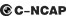## 长安 长安CX308 种颜色可选2012款最低售价：6.38 万元起

4600(mm)1800(mm)1475(mm)##### 配置亮点：
• 胎压监测装置

• ISOFIX儿童座椅接口

• 车身稳定控制(ESC/ESP/DSC等)

• 电动天窗

• 定速巡航

• 后倒车雷达

• 真皮座椅

• GPS导航系统

• 氙气大灯

• 后视镜加热长安 长安CX30 在售车型

排量 车型 厂商指导价 本地最低报价 购车工具
1.6L 三厢 1.6L 手动舒适型 停产停售 5挡手动 6.38万 6.38万

询底价+对比
三厢 1.6L 手动豪华型 停产停售 5挡手动 6.98万 6.98万

询底价+对比
三厢 1.6L 手动豪华低碳版 停产停售 5挡手动 7.28万 7.28万

询底价+对比

长安 长安CX30 经销商

查看更多 >>
更多 >>

本地降价排名

### 长安 长安CX30 动力加速

长安CX30 0-100公里加速时间分布在 0.0-秒 属于 超跑级

动力级别 加速时间 车型

长安 长安CX30 视频

长安 长安CX30 新闻资讯

# 长安星空之旅自由正在路上

试驾评测 超过9396次关注

7日上午9时星空探索团成员从西安市大唐西市博物馆出发，开始了一天的行程。摄像机记下来了CS星空之旅一路上发生的事情，穿梭在这个写满历史的名都，徜徉在经济发展...

# 把实用放在首位 测试长安CX30 1.6MT

评测 超过4921次关注

长安汽车进入家用轿车领域后接连推出了志翔、悦翔、奔奔i、奔奔MINI等车系，产品覆盖了微型、小型、紧凑型三个级别，今天我们测试的这款CX30是在去年五月底正式上市...

# 何必要买小型车 8万元自主紧凑型车推荐

试驾评测 超过4166次关注

提到CX30可能有些车友并不熟悉，甚至会在脑中闪过一些其他品牌的车型。如果您也是这样那也并不奇怪，因为相比于造车，长安在市场宣传方面似乎还有所欠缺，这也使得...

# 2010款长安CX30保养解析 小保养170元

技术 超过5358次关注

长安CX30是长安汽车推出志翔以后的第二款紧凑型轿车，目前志翔车型的产量减小，CX30即成为了长安紧凑型车的主打产品。在我国自主汽车品牌中，长安汽车质量和口碑一...

# 长安2012款CX30三厢上市 售6.38-7.28万

新闻 超过3313次关注

近日获悉，长安汽车2012款CX30三厢版正式上市，新车共推出三款车型选择，售价区间为6.38-7.28万元，相比老款同型号车型售价有所降低。

# 预计7-11万元 长安CX30将于近期上市

新闻 超过2909次关注

近日有消息显示，长安全新两厢新车CX30将于近期正式上市，目前长安志翔的官方售价为7.98～11.58万元，未来CX30的车型售价预计将以此价格作为参考。目前新车已经接受...

# 配1.6/2.0发动机 长安CX30有望下月上市

新闻 超过3816次关注

近日获悉，长安全新两厢轿车CX30有望于即将正式上市，新车即长安志翔的两厢版车型。

猜你喜欢

﻿
• 快速找车
• 选择品牌
• 选择品牌
• A  奥迪
• A  阿斯顿·马丁
• A  阿尔法·罗密欧
• B  宝沃
• B  布加迪
• B  巴博斯
• B  保时捷
• B  宾利
• B  奔驰
• B  宝马
• B  本田
• B  别克
• B  标致
• B  比亚迪
• B  宝骏
• B  北汽制造
• B  北汽新能源
• B  北汽幻速
• B  北汽威旺
• B  北京汽车
• B  奔腾
• B  北汽绅宝
• C  长安
• C  长安商用
• C  长城
• C  昌河
• D  大众
• D  道奇
• D  DS
• D  东南
• D  东风风神
• D  东风风行
• D  东风小康
• D  东风风度
• D  东风
• F  福特
• F  丰田
• F  菲亚特
• F  法拉利
• F  福田
• F  福迪
• F  福汽启腾
• G  观致
• G  广汽传祺
• G  广汽吉奥
• G  GMC
• H  红旗
• H  汉腾汽车
• H  哈弗
• H  哈飞
• H  海格
• H  海马
• H  华颂
• H  黄海
• H  华泰
• H  恒天
• J  吉利汽车
• J  捷豹
• J  Jeep
• J  江淮
• J  江铃
• J  金杯
• J  九龙
• J  金旅
• K  凯翼
• K  凯迪拉克
• K  克莱斯勒
• K  科尼塞克
• K  卡威
• K  开瑞
• L  路虎
• L  林肯
• L  劳斯莱斯
• L  兰博基尼
• L  雷克萨斯
• L  铃木
• L  雷诺
• L  理念
• L  力帆
• L  莲花汽车
• L  猎豹
• L  路特斯
• L  陆风
• M  马自达
• M  MG
• M  MINI
• M  玛莎拉蒂
• M  摩根
• M  迈凯轮
• N  纳智捷
• O  欧宝
• O  讴歌
• O  欧朗
• Q  奇瑞
• Q  起亚
• Q  启辰
• R  日产
• R  荣威
• R  瑞麒
• S  三菱
• S  斯威汽车
• S  萨博
• S  smart
• S  斯柯达
• S  斯巴鲁
• S  思铭
• S  双龙
• S  上汽大通
• S  双环
• T  特斯拉
• T  腾势
• W  沃尔沃
• W  五菱汽车
• W  五十铃
• W  威兹曼
• W  威麟
• X  现代
• X  雪佛兰
• X  雪铁龙
• X  西雅特
• Y  一汽
• Y  英菲尼迪
• Y  英致
• Y  依维柯
• Y  野马汽车
• Y  永源
• Z  众泰
• Z  中华
• Z  中兴
• Z  知豆
• 选择车系
• 选择车系
• 车型对比
• 选择品牌
• 选择品牌
• A  奥迪
• A  阿斯顿·马丁
• A  阿尔法·罗密欧
• B  宝沃
• B  布加迪
• B  巴博斯
• B  保时捷
• B  宾利
• B  奔驰
• B  宝马
• B  本田
• B  别克
• B  标致
• B  比亚迪
• B  宝骏
• B  北汽制造
• B  北汽新能源
• B  北汽幻速
• B  北汽威旺
• B  北京汽车
• B  奔腾
• B  北汽绅宝
• C  长安
• C  长安商用
• C  长城
• C  昌河
• D  大众
• D  道奇
• D  DS
• D  东南
• D  东风风神
• D  东风风行
• D  东风小康
• D  东风风度
• D  东风
• F  福特
• F  丰田
• F  菲亚特
• F  法拉利
• F  福田
• F  福迪
• F  福汽启腾
• G  观致
• G  广汽传祺
• G  广汽吉奥
• G  GMC
• H  红旗
• H  汉腾汽车
• H  哈弗
• H  哈飞
• H  海格
• H  海马
• H  华颂
• H  黄海
• H  华泰
• H  恒天
• J  吉利汽车
• J  捷豹
• J  Jeep
• J  江淮
• J  江铃
• J  金杯
• J  九龙
• J  金旅
• K  凯翼
• K  凯迪拉克
• K  克莱斯勒
• K  科尼塞克
• K  卡威
• K  开瑞
• L  路虎
• L  林肯
• L  劳斯莱斯
• L  兰博基尼
• L  雷克萨斯
• L  铃木
• L  雷诺
• L  理念
• L  力帆
• L  莲花汽车
• L  猎豹
• L  路特斯
• L  陆风
• M  马自达
• M  MG
• M  MINI
• M  玛莎拉蒂
• M  摩根
• M  迈凯轮
• N  纳智捷
• O  欧宝
• O  讴歌
• O  欧朗
• Q  奇瑞
• Q  起亚
• Q  启辰
• R  日产
• R  荣威
• R  瑞麒
• S  三菱
• S  斯威汽车
• S  萨博
• S  smart
• S  斯柯达
• S  斯巴鲁
• S  思铭
• S  双龙
• S  上汽大通
• S  双环
• T  特斯拉
• T  腾势
• W  沃尔沃
• W  五菱汽车
• W  五十铃
• W  威兹曼
• W  威麟
• X  现代
• X  雪佛兰
• X  雪铁龙
• X  西雅特
• Y  一汽
• Y  英菲尼迪
• Y  英致
• Y  依维柯
• Y  野马汽车
• Y  永源
• Z  众泰
• Z  中华
• Z  中兴
• Z  知豆
• 选择车系
• 选择车系
• 选择车型
• 选择车型
• 意见反馈